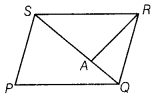# PQRS is a parallelogram, whose area is 180 cm2 and A is any point on the diagonal QS

PQRS is a parallelogram, whose area is 180 cm2 and A is any point on the diagonal QS. Then, the area of ∆ASR - 90 cm2. This statement is true or false. Why?

Given, area of parallelogram PQRS is 180 cm2 and QS is its diagonal which divides it into two triangles of equal areas.
Also, area of ∆ SRQ = 90 cm2Now, A is any point on QS.
∴ Area of ∆ ASR < Area of ∆ SRQ